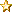###Author Topic: Question about Division  (Read 225 times)

#### Nick_W

• Full Member
•• Posts: 215« on: February 10, 2017, 09:56:18 am »
I know that it was mentioned a while ago that division always returns a Float.

So I have been doing things like this:

Code: [Select]
`Dim Value As Integer..... stuffValue = ((Value + List(currentsample)) / 2).ToInteger()`
everywhere. Now I have to say this seems to be odd behavior, as most languages would simply do integer arithmetic in this case. If I were to do this:

Code: [Select]
`Value = ((Value + List(currentsample)) / 2.0).ToInteger()`
I would expect to have to cast to an integer, as 2.0 is explicitly a Float, and so Value etc. would usually be promoted to a Float.

This means that common constructs like:

Code: [Select]
`Value /= 2`
Don't work as Value is an Integer and the division returns a float (but Value *= 2, +=, -= etc do work - this seems inconsistent).

Looking at the documentation, it gives two division operators (never noticed that before!).

This is what the documentation says:

Quote
Shared Operator /(x As Integer, y As Integer) As Integer
Shared Operator \(x As Integer, y As Integer) As float

So according to the documentation, / should return an Integer, and \ should return a float. In fact, having tested this, it is the opposite way round.

So this does work:

Code: [Select]
`Dim Value As Integer..... stuffValue = (Value + List(currentsample)) \ 2`
And Value \= 2 works too!

I would really like to go through my code and remove all the unneeded type casting, but I'm not sure which is the right form. The documentation does not agree with the compiler.

So which is right? should I refactor my code, or will the compiler be fixed and I can just take out all the type casts as is (in the future)?

Thanks,

#### mc-John

• Global Moderator
• Full Member
•• Posts: 212